American Journal of Theoretical and Applied Statistics
Volume 5, Issue 6, November 2016, Pages: 334-341

Modeling Extremal Events: A Case Study of the Kenyan Public Debt

Josephat Onchangwa Motonu1, *, Anthony Gichuhi Waititu2, Joseph Kyalo Mung’atu2

1Parliamentary Budget Office, Parliament of Kenya, Nairobi, Kenya

2Department of Statistics and Actuarial Science, Jomo Kenyatta University of Agriculture and Technology, Nairobi, Kenya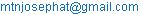(J. O. Motonu)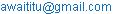(A. G. Waititu)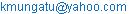(J. K. Mung’atu)

*Corresponding author

Josephat Onchangwa Motonu, Anthony Gichuhi Waititu, Joseph Kyalo Mung’atu. Modeling Extremal Events: A Case Study of the Kenyan Public Debt. American Journal of Theoretical and Applied Statistics. Vol. 5, No. 6, 2016, pp. 334-341. doi: 10.11648/j.ajtas.20160506.11

Received: September 14, 2016; Accepted: September 23, 2016; Published: October 14, 2016

Abstract: Kenya’s public debt is sharply increasing and there are fears that in the long run, the situation in the country may, perhaps, be gravitating towards the boundaries of debt distress. This has been occasioned by the ever rising fiscal deficit as a result of high expenditure appetite and poor performance of tax revenue. In addition to that is the recent surge in mega infrastructure development which is anticipated to continue triggering uptake, and piling of more public debt. To model this phenomenon, this study has applied the Extreme Value Theory in modeling the public debt where Generalized Pareto Distribution has been used and subsequently, Value-at-Risk determined. Generally, the differenced debt stock data has been modeled by fitting the Generalized Pareto Distribution and a debt sustainability threshold has been determined as 1.263. This is interpreted to imply that the prevailing year's borrowing should not occasion a rise in public debt beyond 26.3 per cent of the previous year's level. Specifically, both the unconditional and conditional Value-at-Risk has been ascertained as 1.263 and 0.957 respectively, at α = 0.05 level of significance, which is the maximum tolerable debt limit. Further, by applying the loss function, it has been established that among the two methods, conditional Value-at-Risk is the efficient model for measuring public debt risk, connoting that at α = 0.05, the current year's borrowing, say, should occasion a public debt reduction by 4.27 per cent from the previous one for the country to vacillate within the debt sustainability realms. Finally, it is recommended that a further study be conducted by computing and using Net Present Value of debt indicators since the ones used in this study are aggregated in nominal terms.

Keywords: Debt Distress, Fiscal Deficit, Generalized Pareto Distribution, Value-at-Risk, Loss Function, Debt Sustainability, Net Present Value

1. Introduction

The public debt in Kenya is increasing at an alarming rate and there are fears that eventually, the country may contract debts that it will not be capable to properly service, at the detriment of socio-economic prosperity and well being of future generations. For quite some time now, there has been conspicuous spotlight on this incident. Significantly,  has studied the problem of public debt especially in Africa. From the study, it is deciphered that the debt predicament is easily traceable back to the three main epochs of oil price shocks thus far. For instance, it has been argued that the first epoch of oil price shock of late 1960s to early 1970s led to an increase in commodity prices which in turn triggered an upsurge in public expenditure particularly on social and physical infrastructure. The second aeon of the shock was experienced in the late 1970s to early 1980s and is believed to have occasioned a hike in real interest rates in the developed economies, thereby leading to a rise in the burden of debt servicing, a burden borne by developing countries such as Kenya. The third such episode happened in the late 1980s and early 1990s whose striking outcome was the debt rescheduling through the Paris and London Clubs.

Also, a study by  observes that external indebtedness is major problem that Sub-Saharan Countries are facing currently. It postulates that there is a more severe issue which relates to the implication and sustainability of the huge public debt. This is the mortgaging the aspirations of the future generations, on top of it being a weigh down to the present generation; notwithstanding Africa’s endowment in terms of both human and natural resources. The study further observes that with regards to Africa’s debt on overall, debt servicing burden exceeds national income in some incidences. Also, the ratio of debt to national income is on the upward trajectory coupled with debt serving that is slowly surpassing export value. [3,4] have also studies issues of debt dynamics.

In their Medium Term Debt Strategies, countries often rely on the analytical tool known as the Debt Sustainability Analysis for public debt management. According to  the interpretation of this tool is somewhat supple in the sense that it considers the country’s specific features such as debt stock; debt policy track record; debt to Gross Domestic Product ratio, debt to export ratio, debt to revenue ratio, debt service to export ratio and debt service to revenue ratio; some of whose sustainability thresholds are, in a considered opinion, very high and easy to achieve. Just to illustrate, the debt to Gross Domestic Product ratios in Kenya is currently given a sustainability threshold of 74% by the World Bank. Other indicators are such as such as the debt service to revenue ration with a threshold of 30% whereas Kenya's stands at 29.7%. Present Value of debt to Gross Domestic Product ratio has a threshold of 300% while Kenya has 243%. However, even with the flexibility of the Debt Sustainability Analysis tool, the country still appears to be on the edge of tipping to debt un-sustainability boundaries.

Therefore, there is a compelling desire to model the public debt in Kenya by applying robust statistical techniques capable of determining debt threshold as well as modeling the debt Value-at-Risk. Thus, this has necessitated the choice of applying the Extreme Value Theory in this study. The results arising out of this technique is likely to effectively facilitate determination of the optimum public debt threshold and further, model exceedances over the optimum debt threshold. The results will be utilized to inform the country discourse with regard to crucial debt policy decisions for prudent debt management and also act as a key instrument in handling future public borrowing.

Various scholars have applied the statistical techniques of extremes in their studies. For example,  estimated the extreme value at risk in the Rwanda Exchange Rate. The shape and scale parameters of the Generalized Pareto Distribution (GPD) were estimated using the method of Maximum Likelihood Estimation from the daily exchange rate series data and the Value-at-Risk was calculated for independent and identically distributed standard residuals. The study compared the Extreme Value Theory approach and the Generalized Auto-Regressive Conditional Heteroskedasticity model and established that the two models compare very well under the independent and identically distribution residual assumptions.

Another study by  applied Extreme Value Theory (EVT) in the estimation of Value-at-Risk (VaR) in the Kenyan stock market. They pointed out that quantification of VaR using EVT has the ability to estimate observations at the extreme quantiles. The study used Barclays Bank data from the Nairobi Securities Exchange and observed that Peak-Over-Threshold (POT) model of EVT and the Generalized Pareto Distribution (GPD) captured the rare events hence, making it a robust method of estimating the Value-at-Risk. [8,9,10] have also applied EVT in various studies of extremal events.

This study has applied the Extreme Value Theory to estimate the Value-at-Risk in modeling extremal events problems. However, arising from the existing literature, this study has attempted to focus on the application of the technique to model the public debt phenomenon in Kenya and also determine the efficiency of Value-at-Risk estimates arising from both the unconditional and conditional approaches.

Indeed, this study has made an effort to accomplish the objective of modeling the optimum absorption of public debt in Kenya using the Extreme Value Theory. Further, the focus has been to model public debt exceedance over optimum threshold using both the unconditional and conditional Value-at-Risk and subsequently, a determination as to which of the two approaches yields an efficient VaR measure.

2. Methodology

2.1. Theoretical Review of the Extreme Value Theory

The fundamental statistics of Extreme Value Theory is attributable to the earliest work of . Hitherto, there has been further work on various aspects of the famous "Fisher-Tippet Theorem" by  among other notable contributions.

According to , the Fisher – Tippett Theorem specifies the form and the limit distribution for the centred and normalized maxima or minima. The theorem postulates that for a sequence {Xn}, there exist norming constants cn > 0 and dn ϵ and some non-degenerate density function, H; then cn-1 (Mn - dn) →d H, H must belong to one of the three possible limit laws of extreme value distribution which are:

Fréchet: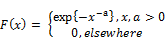(1)

Weibull: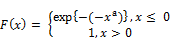(2)

Gumbel: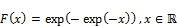(3)

H is referred to as the Generalized Extreme Value (GEV) distribution and is given as:

F(x; µ, σ, ξ) =exp {-[1+ ξ(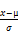)]-1/ξ}            (4)

for 1+ ξ (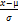) > 0, where µ ϵ ℝ is the location parameter, σ > 0 is the scale parameter and ξ ϵ ℝ is the shape parameter. Extremes happen "near" the upper end (or lower end) of the support of the distribution, hence, the asymptotic behaviour of the maxima, Mn (or minima, M1) must be related to the density function, say, F ; in its tail near the end point. Accordingly, if we consider the right tail, then the right end point is defined as:

XF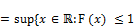}                 (5)

Since the maxima is non decreasing in the sample, then it converges almost surely to the right end point as n → ∞

The three distributions (Fréchet, Weibull and Gumbel) are known as max-stable distributions and for n>2, they satisfy the identity:

Max(x1, x2, ..., xn) = d cn.x + dn                  (6)

for cn > 0 and dn ϵ

It is known that the Maximum Domain of Attraction of Weibull distribution has a finite right end point. Hence, it may not be the best in modeling extremal events and thus, the distributions with infinite right end points such as Fréchet and Gumbel, are preferred.

2.2. Peak over Optimum Threshold

In modeling the excess distribution over a high threshold, the Generalized Pareto Distribution (GPD) is generally chosen as the natural model. It has the density function: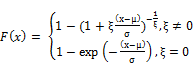(7)

Where: x ≥ 0, σ > 0;≥0; 0 ≤ x ≤in the case where< 0.

The excess distribution over threshold is given as

Fu (x) = Pr(x-u ≤ x|x > u) =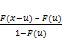(8)

Therefore, of interest here is the probability that a public debt stock which has hitherto been sustainable for u years becomes unsustainable in the period (u, u + x].

Generally, if X has a GPD density function (F = Gξ,β), then the excess density function is: Fu(x) = Gξ,β(u) (x), where β(u) = β +u. This implies that the excess distribution remains a GPD with the same shape parameter, ξ whereas the scale parameter β is growing linearly with the threshold u. Thus, the mean excess function is:

e(u) = E(x-u|x>u) =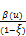=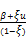, ξ<1              (9)

Clearly, the mean excess function is linear in the threshold u; and this is one of the GPD characterization properties.

2.3. The Pickands-Balkema-de Haan Theorem

The theorem  and  shows that under the Maximum Domain of Attraction (MDA) conditions, we can find a positive measurable function β (u) such that: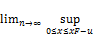|Fu(x) - Gξ,ᵦ(u)(x)| = 0          (10)

iff F ϵ MDA(Hξ), ξ ϵ

Thus, distributions for which normalized maxima converges to GEV distribution, constitute a set of distributions for which excess distribution converges to GPD as the threshold is enhanced. Moreover, the shape parameter of the limiting GPD for the excess is the same as the shape parameter of the limiting GEV distribution for the maxima. This is the canonical distribution for modeling excesses over a high threshold.

The importance is stressed of the contribution made by . A major bottleneck is the choice of u since the theory gives no guidance thus far. Nonetheless, the sample mean plot is usually expected to be linear where an upward trend signifies a GPD model with ξ > 0; horizontal plot is for a GPD model with ξ = 0 and a downward trend signifies a GPD with ξ < 0.

2.4. Unconditional Value-at-Risk

VaR is a single estimate of an amount by which a country’s position in a risk category could decline due to debt movements during a given period. Consider debt stock dt, suppose that the ith exeedance occurs at time ti. Focusing on dt - u where exceedance u is a certain threshold, according to . The conditional distribution is used to handle the magnitude of exceedances given that the threshold is exceeded. The cumulative distribution is a GPD:

Pr(dt ≤ x+ u | dt > u) =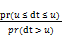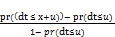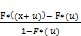(11)

Assuming the baseline time interval is D (operating days or months of a year, say), the conditional approach postulates that the exceeding time and associated debt stock (ti, dti) jointly form a two-dimensional Poisson process with intensity measure given by:

Λ[(D1, D2) * (d, ∞)] =S(d; ξ, µ, σ)        (12)

Where, S(d; ξ, µ, σ) = [1 – ξ (d – σ)/ µ]+1/ξ, 0 ≤ D1 ≤ D2 ≤T; d > u; µ > 0; ξ, σ are parameters and [x]+ = max(x,0)

The occurrence of exceeding threshold is proportional to the length of time interval [D1, D2] and the probability is governed by the survival function which is used to denote the probability of exceedance. Therefore,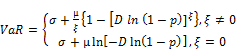(13)

D is the baseline time interval used in estimation for instance, D = 252 or 12 for operating days or months in a year respectively.

2.5. Conditional VaR

This concept postulates that, µ and σ are time varying and are linear functions of explanatory variables; xt = (x1t, x2t, ... xvt)T prior to t. These are variables such as ordinary revenue to debt, debt service to ordinary revenue, export value to external debt and external debt service to export value ratios which are used to estimate model parameters, and we assume that:t = λ0 + λ1x1t + ... + λvxvt ≡ λ0 + λTxt

ln(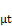) = δ0 + δ1x1t + ... + δvxvt ≡ δ0 + δTxt

σt = γ0 + γ1x1t + ... + γvxvt ≡ γ0 + γTxt             (14)

If λ = 0, then the shape parametert = λ0, which is time invariant. When ξ, µ and σ are time varying, then we have an inhomogeneous Poisson process. The intensity measure then becomes:

Λ [(D1, D2) * (d, ∞)] =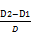[1 – ξt(d – σt)/ µt]+1/ξt, d > u  (15)

The likelihood function is:

L = (∏ D-1g(rti; ξti, µti, σti) * exp [-D-1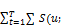ξti, µti, σti)]  (16)

Therefore, given {dt, xt |t=1, ..., T}, the threshold u* and the baseline time interval D, the parameters the in explanatory variables equations have are estimated by maximizing the logarithms of the likelihood function.

2.6. Efficiency of VaR Estimate

According to ; the general form of the loss function is given as: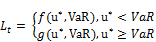(17)

where: f(x,y) and g(x,y) are function such that f(x,y) ≥ g(x,y) for a given y and u* refer to exceedances. The numerical scores are constructed with a negative orientation such that lower values of Lt are preferred because exceedances are allocated higher score than non-exceedances. Therefore, from the viewpoint of the regulator (in this case the Legislature or Cabinet in the Kenyan context), to get a single value;(18)

Ideally, the best model among the unconditional VaR and Conditional VaR is the one that will have the lowest value of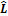. Therefore, loss function can be implied by either the binomial method as: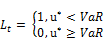(19)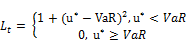(20)

The calculation for the two approaches, unconditional and conditional Value-at-Risk, is done by varying tail probabilities in order to generate different VaR estimates to be used in calculation of the loss function, and ultimately the efficient VaR estimate determined.

3. Results and Discussion

3.1. Exploratory Data Analysis

3.1.1. Time Series Plot for Debt Indicators and Ratios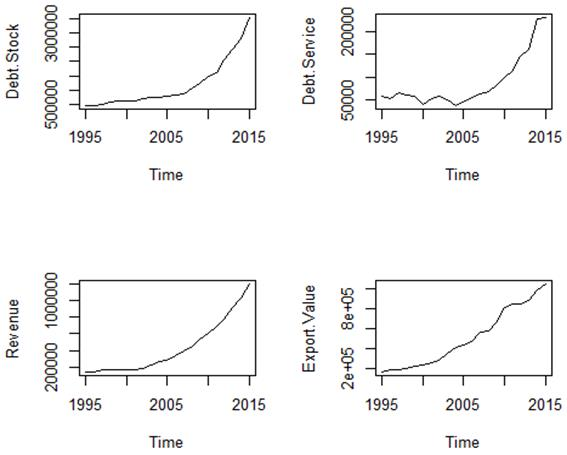(a)(b)

Figure 1. Time series plots. Plot (a) is for debt stock, debt service, revenue and export service and (b) is for the following ratios: ordinary revenue to debt, debt service to ordinary revenue, export value to external debt and external debt service to export value. It is noted that both (a) and (b) exhibit trends that may need to be removed to make the data stationary.

3.1.2. Differenced series for Debt Indicators and Ratios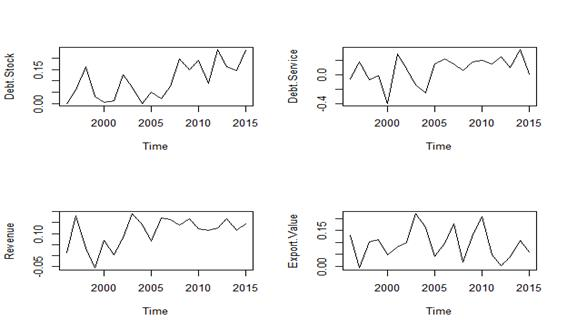(a)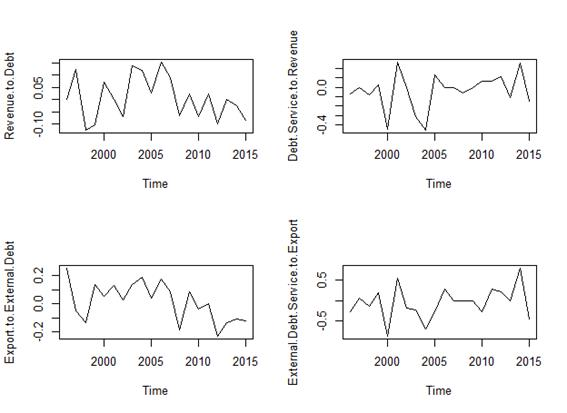(b)

Figure 2. Time differenced series plots. Plot (a) is for debt stock, debt service, revenue and export value and (b) is for the following ratios: ordinary revenue to debt, debt service to ordinary revenue, export value to external debt and external debt service to export value. The trend has been eliminated by differencing the data and it is now stationary.

3.1.3. Normality Test Using Histogram and Q-Q plot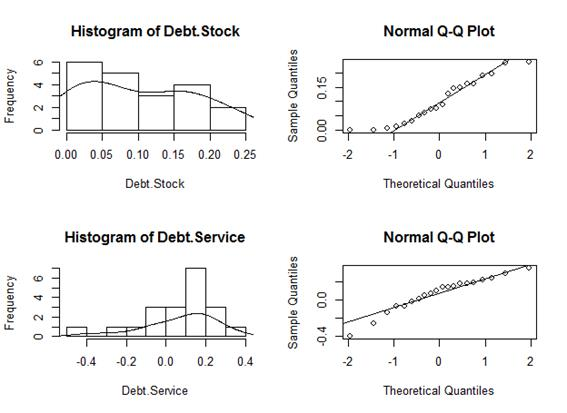(a)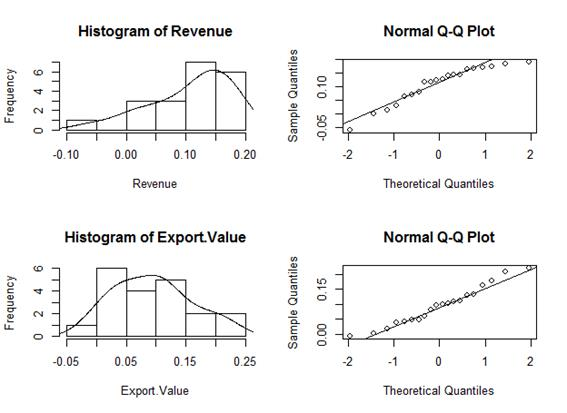(b)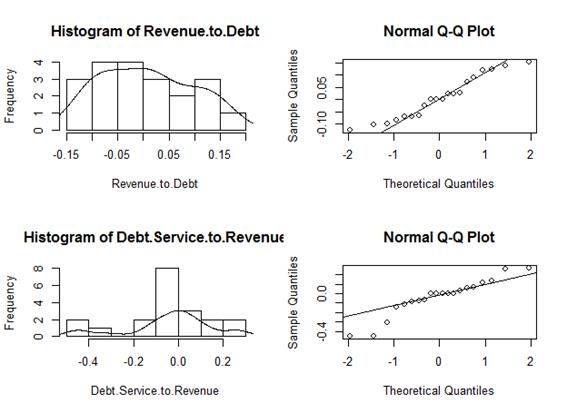(c)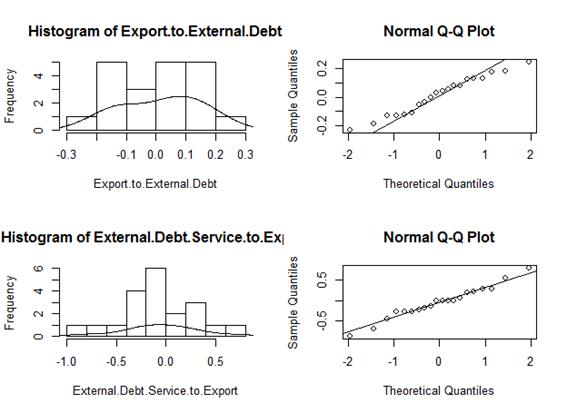(d)

Figure 3. Histogram and Q-Q plots for differenced series. Plots (a) and (b) represent debt stock, debt service, revenue and export value whereas plots (c) and (d) represent the ratios: ordinary revenue to debt, debt service to ordinary revenue, export value to external debt and external debt service to export value. It is observed that in all the 8 indicators, the histograms exhibit skewness either to the left or to the right, hence, an evidence of a heavy tailed distribution. For the normal Q-Q plots, a 45 degree reference line is plotted. Ideally, when all the points lie along this line, then it is concluded that the empirical data comes from the normal distribution. A departure of the points from this reference line, as depicted by these indicators under considerations, is as further evidence that the data is from a heavy tailed distribution.

3.1.4. Independence Test Using ACF and PACF Plots

Testing for independence entails plotting the auto-correlation function and partial auto-correlation functions to check for the Auto-Regressive Conditional Heteroskedasticity (ARCH) effects (presence of serial correlation) and test for independence respectively. If for instance, we take an equation dt = μt + at where dt represents the public debt series, μt is the mean equation and at is of the innovation or shock of public debt at time t.

Now, by letting at = dt – μt be the residuals of the mean equation, then the squared series at2 is used to check the existence of the ARCH effects.

The plots are implemented as shown in figure 4.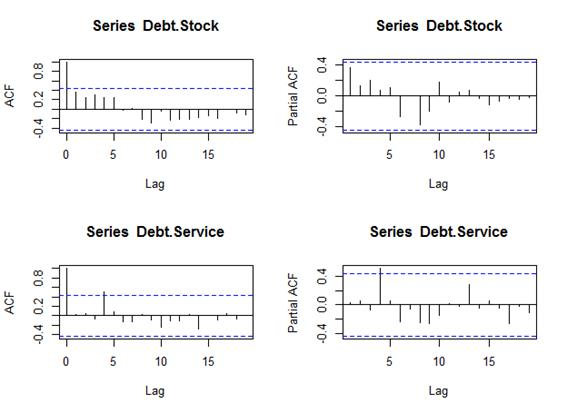(a)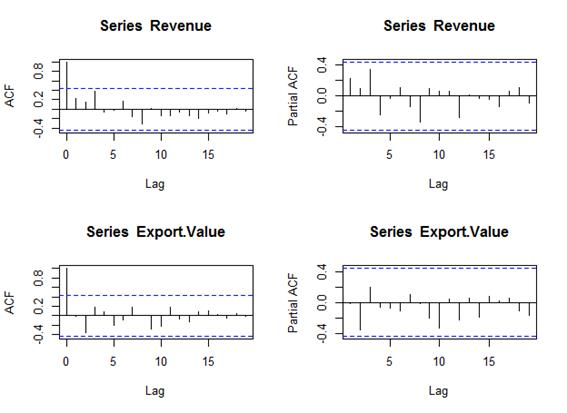(b)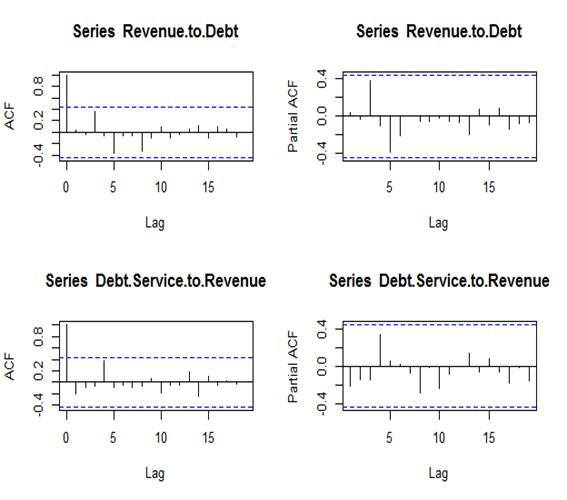(c)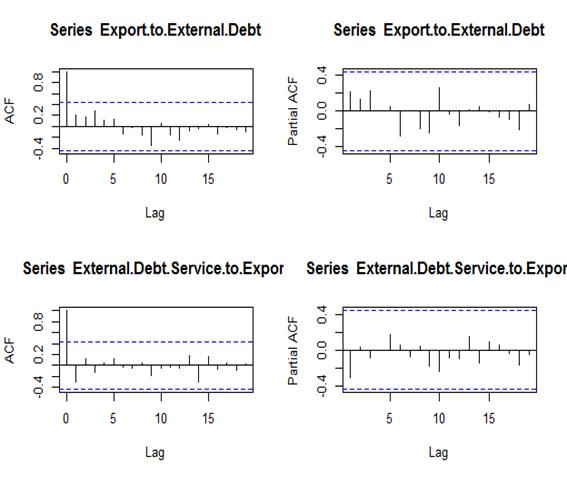(d)

Figure 4. ACF and PACF for differenced series: Plot (a) and (b) represent debt stock, debt service, revenue and export value whereas plots (c) and (d) are for the ratios: ordinary revenue to debt, debt service to ordinary revenue, export value to external debt and external debt service to export value respectively. Clearly, there are no noteworthy spikes protruding from the confidence bands at lag 20 for both the ACF and PACF graphs. Therefore, this implies that there is no significant serial correlation and that there is also no serial dependence. Hence, leading to the conclusion that there are no ARCH effects and there exist no evidence against the independence assumption.

3.2. Threshold Determination

The threshold is determined using the R function; find Threshold, which finds a threshold by fitting differenced series for debt stock, such that a given number of exceedances fall above. In the event that there happens to be a tie in the data, then in such a case a threshold is determined in a manner that at least the specified number of exceedances fall above. The following is the result of fitting a GPD model to determine the threshold.

Table 1. Threshold determination.

 Number Parameter Value 1 Threshold 0.2333263 2 ξ - 1.1520949 3 β 0.3734691

From table 1, the threshold is 0.233. Clearly, loge (Yt /Yt-1) = 0.233, where Yt and Yt-1 represent the public debt in year t and t-1 respectively. This then leads to (Yt/Yt-1) = 1.263, implying that the public debt threshold is 1.263. This means that the current year's borrowing should not occasion public debt to rise beyond 26.3 per cent of the previous year one.

To estimate the Generalized Pareto Distribution (GPD) model parameters, the shape parameter, ξ = -1.152 and the scale parameter, β = 0.273. Since ξ < 0, then the GPD model simplifies to Pareto type II distribution.

3.3. Unconditional Value-at-Risk (VaR)

The implementation of the unconditional Value-at-Risk makes use of the R function VaR which returns a numeric value for the differenced debt stock time series data.

Table 2. Unconditional VaR Estimates.

 Tail Probability 99% 98% 97% 96% 95% VaR 0.238 0.238 0.238 0.238 0.233

From the result in table 2, loge (Yt /Yt-1) = 0.233, at α = 0.05, where Yt and Yt-1 represent the public debt ratios matrix in year t and t-1 respectively. This yields (Yt /Yt-1) = 1.263, implying that the public debt Value-at-Risk is 1.263, which means that the maximum tolerable public debt limit in say, the current year is 1.263 times that of the previous one.

3.4. Conditional VaR

The implementation of the conditional Value-at-Risk makes use of the R function CVaR which returns a numeric value for a the differenced debt ratios time series data which is a vector matrix containing ratio: Ordinary Revenue to Debt, Debt Service to Ordinary Revenue, Export to External Debt Service and External Debt Service to Export Value.

Table 3. Conditional VaR Estimates.

 Tail Probability 99% 98% 97% 96% 95% VaR -0.026 -0.032 -0.037 -0.040 -0.044

The result in table 3 indicates that loge (Yt/Yt-1) = -0.044, at α = 0.05, where Yt and Yt-1 represent the public debt ratios matrix in year t and t-1 respectively. This yields (Yt/Yt-1) = 0.957, implying that the public debt Value-at-Risk is 0.957, which is the maximum tolerable public debt limit in the prevailing year compared to the previous. This is interpreted to mean that the current year's borrowing should occasion a public debt reduction by 4.27 per cent from the previous one.

3.5. Efficiency of VaR Estimate

In order to determine which of the model between unconditional and conditional Value-at-Risk is efficient, we make use of the Lopez’s Loss Function which is implemented as shown in table 4.

Table 4. Efficiency of VaR estimate.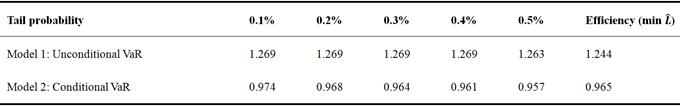From table 4, it is noted thatfor model 1 is 1.244 and that of model 2 is 0.965. Therefore, the minimum is model 2 for the conditional VaR, implies that this is the efficient model that can be used to calculate the Value-at-Risk in modeling the public debt.

4. Summary, Conclusion and Recommendation

4.1. Summary

This study has modeled the Kenyan public debt by applying extremal events techniques. Part 1 presents the introduction, background information and literature review; part 2 has documented the methodology used in the study; part 3 presents the results of the key findings of the study.

Data was initially explored to test the validity of key assumptions underlying the modeling environment. First, a time series plot exhibited a presence of trend in all the parameters under consideration and was removed by differencing the series. Secondly, the data was tested for normality using Histogram and Q-Q plots. The normality assumption was rejected in favour of heavy tailed distributions. Finally, tests for independence of data were achieved by plotting the Auto-Correlation Function and Partial Auto-correlation Functions and it was concluded that there existed neither significant serial correlation nor dependence.

Considering the objectives of the study, the Kenyan public debt threshold has been determined as 1.263 implying that the current year's borrowing should not occasion public debt to rise beyond 26.3 per cent of the previous year's level.

Then, the unconditional Value-at-Risk (VaR) has been determined as 1.263 at α = 0.05. This implies that the public debt Value-at-Risk is 1.263, which means that the maximum tolerable public debt limits in, say, the current year is 1.263 times that of the previous year.

Further, the conditional Value-at-Risk has been established as 0.957 at α = 0.05, implying that the public debt Value-at-Risk is 0.957, which is the maximum tolerable public debt limit in the prevailing year compared to the previous. In other words, the current year's borrowing should occasion a public debt reduction by 4.27 per cent from the previous one.

Finally, this study has ascertained that among the two VaR models, the conditional VaR is the efficient one in measuring the risk associated with modeling the public debt in Kenya.

4.2. Conclusion

In conclusion therefore, this study has established that the public debt threshold of 1.263 implying that the current year's borrowing should not occasion public debt to rise beyond 26.3 per cent of the previous year's level.

The measure of the Value-at-Risk is 0.957 given by the conditional VaR at α = 0.05 level of significance. This means that the public debt Value-at-Risk is 0.957, which is the maximum tolerable public debt limit in the prevailing year compared to the previous. Ideally, the current year's borrowing should occasion a public debt reduction by 4.27 per cent from the previous one.

4.3. Recommendation

It is recommended that a further study be conducted by computing and using the Net Present Value of the Debt Stock as well as for the other parameters used in this study which are: Gross Domestic Product, Revenue, Debt Service and Export Value. This is because the data used in this study is aggregated on nominal terms and it will be of interest to establish the effect of using the Present Value measure. It is further recommended that, from the public finance practitioner's perspective, deliberate measures should be instituted geared towards enhancing local tax revenue mobilization in order to significantly reverse the growing fiscal deficit. This is in a bid to try to dampen the borrowing appetite and ultimately spur economic growth in the long run.

References

1. Alemayehu, F. G. (2003). The historical origin of African debt crisis. Eastern Africa Science Research Review, 19.
2. Lekomola, M. (2010). The African debt dilemma: An overview of magnitude, causes, effects and policy options. Journal of American Sciences, 6.
3. Reinhart, C. and Rogoff, K. (2011). From financial crash to debt crisis. American Economic Review, 101.
4. Panizza, U. (2008). Domestic and external public debt in developing countries. Policy Paper 188, UNCTAD.
5. Sarwaat, J., Xavier, M., Peter, A. C., and Sean, N. (2014). Staff guidance note on the fund’s engagement with small developing states. Policy Paper, International Monetary Fund, P.O. Box 92780, Washington D.C. 20090.
6. Ntawihebasenga, J. D., Mwita, J. K., and Mung’atu, J. K. (2014). Estimation of extreme value at risk in Rwanda exchange rate. European Journal of Statistics and Probability, 2: 14-22.
7. Koima, J. K., Mwita, P. N., and Nassiuma, D. K. (2013). An application of extreme value theory in the estimation of value-at-risk in Kenya stock market. Int. J. Cur. Tr. Res, 2: 276-284.
8. Valayoudoum, M, Bechir, R., and Abdelwahed, T. (2009). Extreme value theory and value-at-risk: Application to oil market. Energy Economics, (31).
9. Manfred, G. and Elvis, K. (2006). An application of extreme value theory for measuring financial risk. Computational Economics, 27(1): 1-23.
10. Ramazan, G. and Faruk, S. (2004). Extreme value theory and value-at-risk: Relative performance in emerging markets. International Journal of forecasting, 20: 287-303.
11. Fisher, R. and Tippet, L. (1928). Limiting forms of the frequency distribution of the largest or the smallest member of a sample. Proc. Cambridge Phil. Soc., 24: 180-190.
12. Davison, A. C. and Smith, R. L. (1990). Models for exceedance over high thresholds. Journal of Royal Statistics Society, B52(3): 393-442.
13. Embrechts, P. Kluppelberg, C., and Mikosch, T. (1997). Modeling Extremal Events for Finance and Insurance. Springer.
14. Pickands, J. (1975). Statistical inference using extreme order statistics. Annals of Probability, 3: 119-131.
15. Balkema, A. and de Haan, L. (1974). Residual life time at great age. Annals of Probability, 3: 792-804.
16. Samuel, K. and Norman, L. J., editors (1992). Breakthroughs in Statistics, volume 1. Springer, New York, New York.
17. Tsay, R. S. (2005). Analysis of Financial Time Series. Wiley, New York.
18. Lopez, J. A. (1999). Methods of evaluating value-at-risk estimates. Federal Reserve Bank of San Francisco Economic Review, 2.

 Contents 1. 2. 2.1. 2.2. 2.3. 2.4. 2.5. 2.6. 3. 3.1. 3.2. 3.3. 3.4. 3.5. 4. 4.1. 4.2. 4.3.
Article ToolsAbstractPDF(542K)